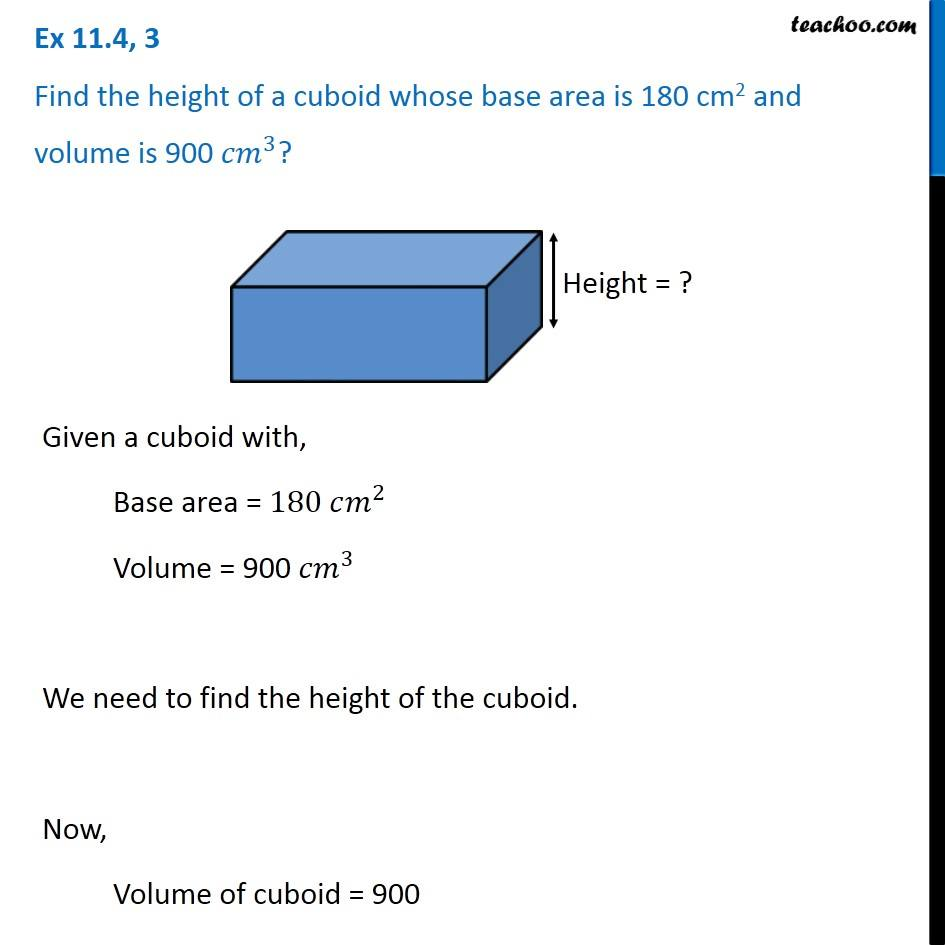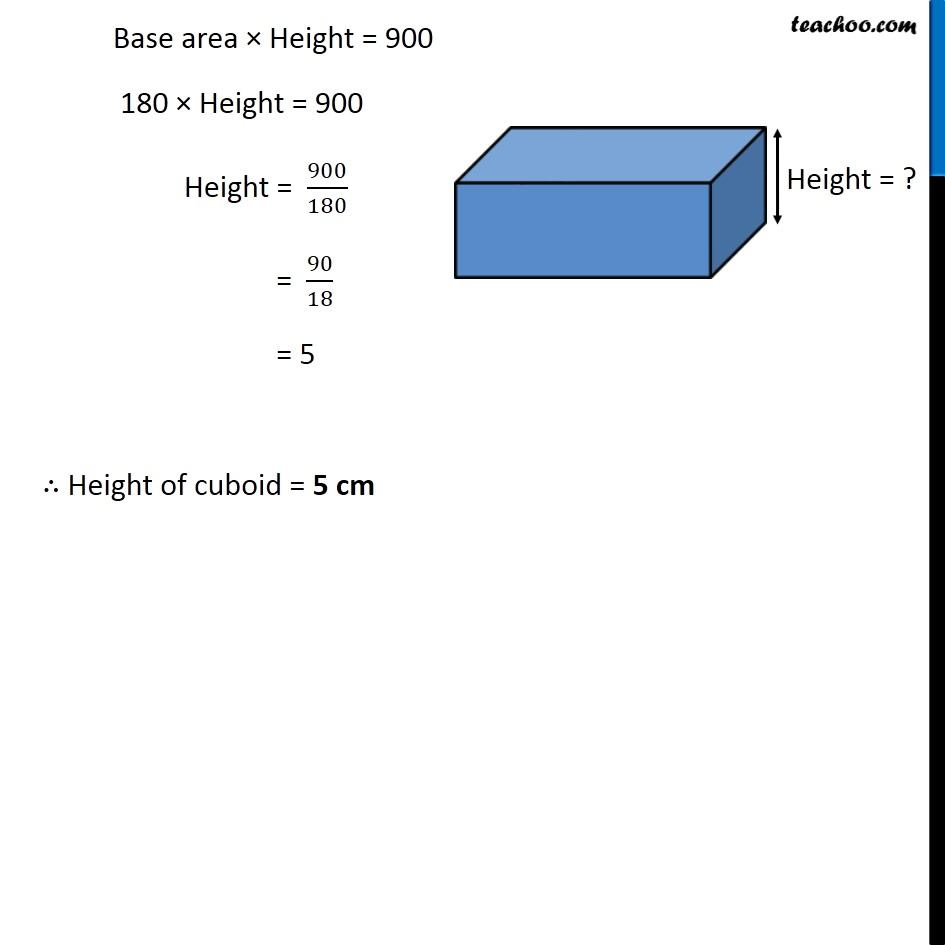Volume of Cuboid

Chapter 11 Class 8 Mensuration
Concept wiseIntroducing your new favourite teacher - Teachoo Black, at only ₹83 per month

### Transcript

Ex 11.4, 3 Find the height of a cuboid whose base area is 180 cm2 and volume is 900 〖𝑐𝑚〗^3? Given a cuboid with, Base area = 180 𝑐𝑚^2 Volume = 900 〖𝑐𝑚〗^3 We need to find the height of the cuboid. Now, Volume of cuboid = 900 Base area × Height = 900 180 × Height = 900 Height = 900/180 = 90/18 = 5 ∴ Height of cuboid = 5 cm